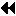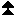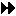## 6.3.3. ExamplesUp: Communication Calls Next: Accumulate Functions Previous: Get

Example We show how to implement the generic indirect assignment A = B(map), where A, B and map have the same distribution, and map is a permutation. To simplify, we assume a block distribution with equal size blocks.

```SUBROUTINE MAPVALS(A, B, map, m, comm, p)
USE MPI
INTEGER m, map(m), comm, p
REAL A(m), B(m)

INTEGER otype(p), oindex(m),   & ! used to construct origin datatypes
ttype(p), tindex(m),      & ! used to construct target datatypes
count(p), total(p),       &
sizeofreal, win, ierr

! This part does the work that depends on the locations of B.
! Can be reused while this does not change

CALL MPI_TYPE_EXTENT(MPI_REAL, sizeofreal, ierr)
CALL MPI_WIN_CREATE(B, m*sizeofreal, sizeofreal, MPI_INFO_NULL,   &
comm, win, ierr)

! This part does the work that depends on the value of map and
! the locations of the arrays.
! Can be reused while these do not change

! Compute number of entries to be received from each process

DO i=1,p
count(i) = 0
END DO
DO i=1,m
j = map(i)/m+1
count(j) = count(j)+1
END DO

total(1) = 0
DO i=2,p
total(i) = total(i-1) + count(i-1)
END DO

DO i=1,p
count(i) = 0
END DO

! compute origin and target indices of entries.
! entry i at current process is received from location
! k at process (j-1), where map(i) = (j-1)*m + (k-1),
! j = 1..p and k = 1..m

DO i=1,m
j = map(i)/m+1
k = MOD(map(i),m)+1
count(j) = count(j)+1
oindex(total(j) + count(j)) = i
tindex(total(j) + count(j)) = k
END DO

! create origin and target datatypes for each get operation
DO i=1,p
CALL MPI_TYPE_INDEXED_BLOCK(count(i), 1, oindex(total(i)+1),   &
MPI_REAL, otype(i), ierr)
CALL MPI_TYPE_COMMIT(otype(i), ierr)
CALL MPI_TYPE_INDEXED_BLOCK(count(i), 1, tindex(total(i)+1),   &
MPI_REAL, ttype(i), ierr)
CALL MPI_TYPE_COMMIT(ttype(i), ierr)
END DO

! this part does the assignment itself
CALL MPI_WIN_FENCE(0, win, ierr)
DO i=1,p
CALL MPI_GET(A, 1, otype(i), i-1, 0, 1, ttype(i), win, ierr)
END DO
CALL MPI_WIN_FENCE(0, win, ierr)

CALL MPI_WIN_FREE(win, ierr)
DO i=1,p
CALL MPI_TYPE_FREE(otype(i), ierr)
CALL MPI_TYPE_FREE(ttype(i), ierr)
END DO
RETURN
END
```

Example

A simpler version can be written that does not require that a datatype be built for the target buffer. But, one then needs a separate get call for each entry, as illustrated below. This code is much simpler, but usually much less efficient, for large arrays.

```SUBROUTINE MAPVALS(A, B, map, m, comm, p)
USE MPI
INTEGER m, map(m), comm, p
REAL A(m), B(m)
INTEGER sizeofreal, win, ierr

CALL MPI_TYPE_EXTENT(MPI_REAL, sizeofreal, ierr)
CALL MPI_WIN_CREATE(B, m*sizeofreal, sizeofreal, MPI_INFO_NULL,  &
comm, win, ierr)

CALL MPI_WIN_FENCE(0, win, ierr)
DO i=1,m
j = map(i)/p
k = MOD(map(i),p)
CALL MPI_GET(A(i), 1, MPI_REAL, j, k, 1, MPI_REAL, win, ierr)
END DO
CALL MPI_WIN_FENCE(0, win, ierr)
CALL MPI_WIN_FREE(win, ierr)
RETURN
END
```Up: Communication Calls Next: Accumulate Functions Previous: Get